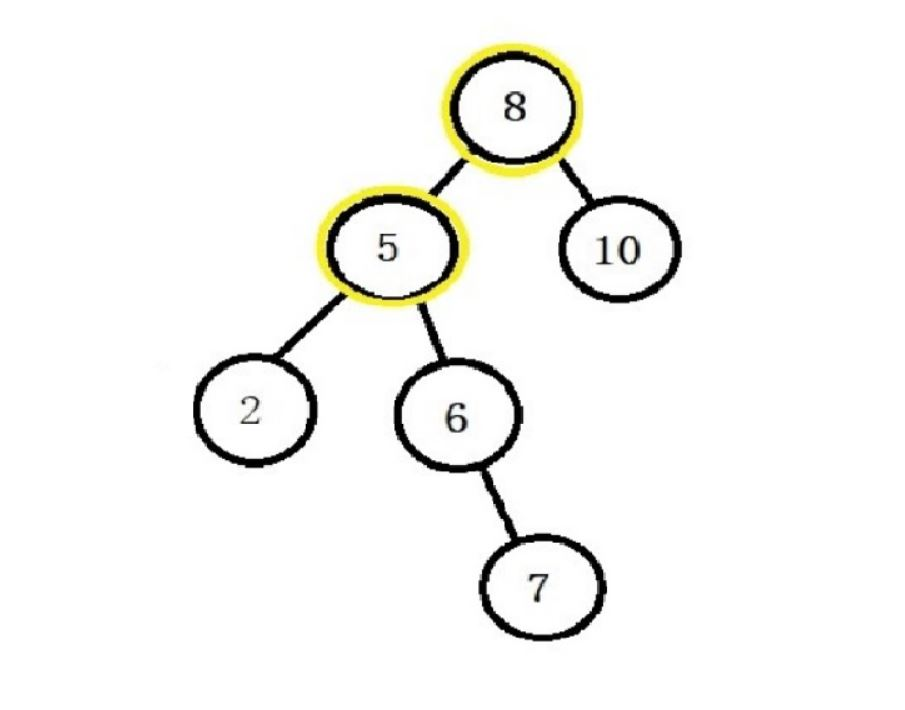New update is available. Click here to update.

# Pair Sum in BST.

Contributed by
Deepanshu_1780
Last Updated: 23 Feb, 2023
Easy0/40
Avg time to solve 15 mins
Success Rate 85 %Share12 upvotes

## Problem Statement

#### A binary search tree (BST) is a binary tree data structure which has the following properties.

``````• The left subtree of a node contains only nodes with data less than the node’s data.
• The right subtree of a node contains only nodes with data greater than the node’s data.
• Both the left and right subtrees must also be binary search trees.
``````
##### Note:
``````1. All the elements of the Binary Search Tree are unique.
2. You can’t use the same node value/element of BST twice.
``````
Detailed explanation ( Input/output format, Notes, Images )##### Sample Input 1:
``````2
8 5 10 2 6 -1 -1 -1 -1 -1 7 -1 -1
13
8 5 10 2 6 -1 -1 -1 -1 -1 7 -1 -1
19
``````
##### Sample Output 1:
``````true
false
``````
##### Explanation for sample input 1:
``````For the first test case, the nodes with values 8 and 5 as shown in the below diagram, gives sum equal to the given target 13. Therefore, the output will be “true” i.e it is possible to find a pair in the given BST having sum equal to ‘K’.

For the second test case, there are no two elements in the given BST such that their sum is equal to the given target ‘K’ = 19.
``````##### Sample Input 2 :
``````2
20 16 -1 12 -1 8 -1 4 -1 -1 -1
12
15 10 20 8 12 16 25 -1 -1 -1 -1 -1 -1 -1 -1
57
``````
##### Sample Output 2:
``````true
false
``````AutocompleteConsole